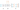← Go BackMarch 26, 20202 min read • IN tech

## Neural Net Problems - Exercise 4

Here are my solutions to exercise 4.

## Implementing Our Network to Classify Digits

### Part 1

#### Question

Write out $a'=\sigma(wa+b)$ in component form, and verify that it gives the same result as the rule, $\frac{1}{1+\exp(- \sum_j w_j x_j - b)}$, for computing the output of a sigmoid neuron.

#### Solution

Let it be stated that I have not yet taken linear algebra at college, so I have very limited experience with it.

Let us say that layer 2 has $2$ nodes and layer 1 has $3$ nodes.

The weights from layer 1 to layer 2 can be expressed as the following ($w_{ji}$, where $j$ is the neuron in the second layer and $i$ is the neuron in the first layer):

$w = \begin{bmatrix} w_{11} & w_{12} & w_{13}\\ w_{21} & w_{22} & w_{23} \end{bmatrix} a = \begin{bmatrix} a_1\\ a_2\\ a_3 \end{bmatrix} b = \begin{bmatrix} b_1\\ b_2 \end{bmatrix}$$wa = \begin{bmatrix} w_{11} a_1 + w_{12} a_2 + w_{13} a_3\\ w_{21} a_1 + w_{22} a_2 + w_{23} a_3 \end{bmatrix}$$wa + b = \begin{bmatrix} (w_{11} a_1 + w_{12} a_2 + w_{13} a_3) + b_1\\ (w_{21} a_1 + w_{22} a_2 + w_{23} a_3) + b_2 \end{bmatrix}$$a' = \sigma(wa + b) = \begin{bmatrix} \sigma((w_{11} a_1 + w_{12} a_2 + w_{13} a_3) + b_1)\\ \sigma((w_{21} a_1 + w_{22} a_2 + w_{23} a_3) + b_2) \end{bmatrix}$

This is exactly the same as $\frac{1}{1+\exp(- \sum_j w_j x_j - b)}$ but computed for both neurons at once with matrices!

Say we wanted to compute the output of the first sigmoid neuron in layer 2.

$a_1' = \sigma(\sum_j w_j a_j + b) = \sigma((w_1 a_1 + w_2 a_2 + w_3 a_3) + b)$

Also, I wanted to note that I found this website called the ml cheatsheet and it has been really useful in describing the mathematic concepts.